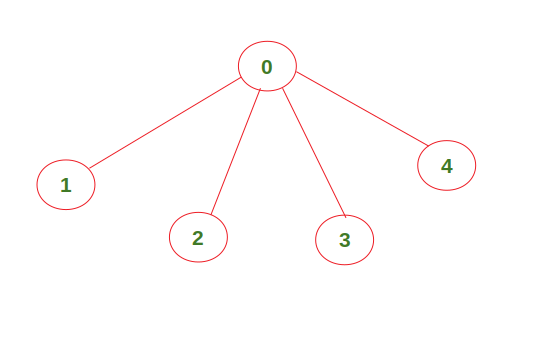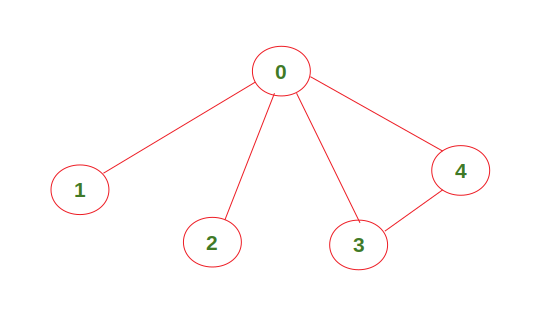Find if an undirected graph contains an independent set of a given size

• Difficulty Level : Medium
• Last Updated : 11 Aug, 2021

Given an undirected graph, check if it contains an independent set of size k. Print ‘Yes’ if there exists an independent set of size k. Print ‘No’ otherwise.

Independent Set: An independent set in a graph is a set of vertices that are not directly connected to each other.

Example 1:

Input : K = 4,
graph = [[1, 1, 0, 0, 0],
[1, 1, 1, 1, 1],
[0, 1, 1, 0, 0],
[0, 1, 0, 1, 0],
[0, 1, 0, 0, 1]]
Output : YesThe above graph contains an independent set of size 4 (vertices 1, 2, 3, 4 are not directly connected to each other). Hence, the output is ‘Yes’.

Example 2:

Input : K = 4,
graph = [[1, 1, 0, 0, 0],
[1, 1, 1, 1, 1],
[0, 1, 1, 0, 0],
[0, 1, 0, 1, 1],
[0, 1, 0, 1, 1]]
Output : NoThe above graph doesn’t contain an independent set of size 4. Hence, the output is ‘No’.

Approach:

• Initialize a variable sol with boolean False value.
• Find all the possible sets of vertices of size K from the given graph.
• If an independent set of size k is found, change the value of sol to True and return.
• Else continue checking for other possible sets.
• In the end, if sol is True, print ‘Yes’ else print ‘No’.

Below is the implementation of the above approach:

C++14

 // C++ code to check if a given graph // contains an independent set of size k #include using namespace std;   // Function prototype bool check(int[], vector &, int);   // Function to construct a set of given size k bool func(int graph[], vector &arr,           int k, int index, bool sol[]) {     // Check if the selected set is independent or not.     // Change the value of sol to True and return     // if it is independent     if (k == 0)     {         if (check(graph, arr, arr.size()))         {             sol = true;             return true;         }     }     else     {         // Set of size k can be formed even if we don't         // include the vertex at current index.         if (index >= k)         {             vector newvec(arr.begin(), arr.end());             newvec.push_back(index);             return (func(graph, newvec, k - 1,                                 index - 1, sol) or                     func(graph, arr, k, index - 1, sol));         }           // Set of size k cannot be formed if we don't         // include the vertex at current index.         else         {             arr.push_back(index);             return func(graph, arr, k - 1,                           index - 1, sol);         }     } }   // Function to check if the given set is // independent or not // arr --> set of size k (contains the // index of included vertex) bool check(int graph[], vector &arr, int n) {     // Check if each vertex is connected to any other     // vertex in the set or not     for (int i = 0; i < n; i++)         for (int j = i + 1; j < n; j++)             if (graph[arr[i]][arr[j]] == 1)                 return false;     return true; }   // Driver Code int main() {     int graph[] = {{1, 1, 0, 0, 0},                       {1, 1, 1, 1, 1},                       {0, 1, 1, 0, 0},                       {0, 1, 0, 1, 0},                       {0, 1, 0, 0, 1}};     int k = 4;     vector arr; // Empty set     bool sol[] = {false};     int n = sizeof(graph) /             sizeof(graph);     func(graph, arr, k, n - 1, sol);       if (sol)         cout << "Yes" << endl;     else         cout << "No" << endl;     return 0; }   // This code is contributed by // sanjeev2552

Java

 // Java code to check if a // given graph contains an // independent set of size k import java.util.*; class GFG{   static  Vector arr =         new Vector<>();   // Function to cona set of // given size k static boolean func(int graph[][],                     int k, int index,                     boolean sol[]) {   // Check if the selected set is   // independent or not. Change the   // value of sol to True and return   // if it is independent   if (k == 0)   {     if (check(graph,               arr.size()))     {       sol = true;       return true;     }   }   else   {     // Set of size k can be     // formed even if we don't     // include the vertex at     // current index.     if (index >= k)     {       Vector newvec =              new Vector<>();       newvec.add(index);       return (func(graph, k - 1,                    index - 1, sol) ||               func(graph, k,                    index - 1, sol));     }       // Set of size k cannot be     // formed if we don't include     // the vertex at current index.     else     {       arr.add(index);       return func(graph, k - 1,                   index - 1, sol);     }   }   return true; }   // Function to check if the // given set is independent // or not arr -. set of size // k (contains the index of // included vertex) static boolean check(int graph[][],                      int n) {   // Check if each vertex is   // connected to any other   // vertex in the set or not   for (int i = 0; i < n; i++)     for (int j = i + 1; j < n; j++)       if (graph[arr.get(i)][arr.get(j)] == 1)         return false;   return true; }   // Driver Code public static void main(String[] args) {   int graph[][] = {{1, 1, 0, 0, 0},                    {1, 1, 1, 1, 1},                    {0, 1, 1, 0, 0},                    {0, 1, 0, 1, 0},                    {0, 1, 0, 0, 1}};   int k = 4;   boolean []sol = new boolean;   int n = graph.length;   func(graph, k, n - 1, sol);     if (sol)     System.out.print("Yes" + "\n");   else     System.out.print("No" + "\n"); } }   // This code is contributed by Amit Katiyar

Python3

 # Python3 code to check if a given graph # contains an independent set of size k   # Function to construct a set of given size k def func(graph, arr, k, index, sol):           # Check if the selected set is independent or not.     # Change the value of sol to True and return     # if it is independent     if k == 0:         if check(graph, arr) == True:             sol = True             return               else:         # Set of size k can be formed even if we don't         # include the vertex at current index.         if index >= k:             return (func(graph, arr[:] + [index], k-1, index-1, sol) or                     func(graph, arr[:], k, index-1, sol))                   # Set of size k cannot be formed if we don't         # include the vertex at current index.         else:             return func(graph, arr[:] + [index], k-1, index-1, sol)   # Function to check if the given set is # independent or not # arr --> set of size k (contains the # index of included vertex) def check(graph, arr):           # Check if each vertex is connected to any other     # vertex in the set or not     for i in range(len(arr)):         for j in range(i + 1, len(arr)):                           if graph[arr[i]][arr[j]] == 1:                 return False                       return True   # Driver Code    graph = [[1, 1, 0, 0, 0],          [1, 1, 1, 1, 1],          [0, 1, 1, 0, 0],          [0, 1, 0, 1, 0],          [0, 1, 0, 0, 1]]           k = 4 arr = []     # Empty set sol = [False]   func(graph, arr[:], k, len(graph)-1, sol)   if sol == True:     print("Yes") else:     print("No")

C#

 // C# code to check if a // given graph contains an // independent set of size k using System; using System.Collections.Generic;   class GFG{   static List arr = new List();   // Function to cona set of // given size k static bool func(int [,]graph,                  int k, int index,                  bool []sol) {       // Check if the selected set is   // independent or not. Change the   // value of sol to True and return   // if it is independent   if (k == 0)   {     if (check(graph,               arr.Count))     {       sol = true;       return true;     }   }   else   {           // Set of size k can be     // formed even if we don't     // include the vertex at     // current index.     if (index >= k)     {       List newvec = new List();       newvec.Add(index);               return (func(graph, k - 1,                        index - 1, sol) ||               func(graph, k,                    index - 1, sol));     }           // Set of size k cannot be     // formed if we don't include     // the vertex at current index.     else     {       arr.Add(index);               return func(graph, k - 1,                      index - 1, sol);     }   }   return true; }   // Function to check if the // given set is independent // or not arr -. set of size // k (contains the index of // included vertex) static bool check(int [,]graph, int n) {       // Check if each vertex is   // connected to any other   // vertex in the set or not   for(int i = 0; i < n; i++)     for(int j = i + 1; j < n; j++)       if (graph[arr[i],arr[j]] == 1)         return false;       return true; }   // Driver Code public static void Main(String[] args) {   int [,]graph = { { 1, 1, 0, 0, 0 },                    { 1, 1, 1, 1, 1 },                    { 0, 1, 1, 0, 0 },                    { 0, 1, 0, 1, 0 },                    { 0, 1, 0, 0, 1 } };   int k = 4;   bool []sol = new bool;   int n = graph.GetLength(0);       func(graph, k, n - 1, sol);     if (sol)     Console.Write("Yes" + "\n");   else     Console.Write("No" + "\n"); } }   // This code is contributed by Amit Katiyar

Output:

Yes

Time Complexity:where V is the number of vertices in the graph and k is the given size of set.
Auxiliary Space: O(V)

My Personal Notes arrow_drop_up
Recommended Articles
Page :